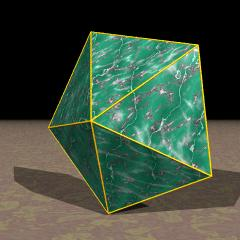Acronym n/d-py Name n/d-gonal pyramid `© ©` Circumradius 1/sqrt[4-1/sin2(πd/n)] Vertex figures [3n]/d, [3,3,n/d] General of army if d=1:   is itself convex if gcd(n,d)=1:   then d=1 provides a topological variant of its general Colonel of regiment (is itself locally convex) Dual (topologically selfdual, in different orientation) Especially tet (n=3, d=1)   squippy (n=4, d=1)   peppy (n=5, d=1)   stappy (n=5, d=2)   hippy (n=6, d=1)   shappy (n=7, d=2)   ogpy (n=8, d=3)

The height formula given below shows that only 2 < n/d < 6 is possible. The maximal height would be obtained at n/d = 2 with upright latteral triangles, the other extremal value n/d = 6 would generate a height of zero.

Incidence matrix according to Dynkin symbol

```oxn/doo&#x   (2<n/d<6)   → height = sqrt(1-1/[4 sin2(π d/n)])
(pt || {n/d})

tip  o.   o.    | 1 * | 4 0 | 4 0
base .o   .o    | * n | 1 2 | 2 1
----------------+-----+-----+----
lace oon/doo&#x | 1 1 | n * | 2 0
base .x   ..    | 0 2 | * n | 1 1
----------------+-----+-----+----
coat ox   ..&#x | 1 2 | 2 1 | n *
base .xn/d.o    | 0 n | 0 n | * 1
```